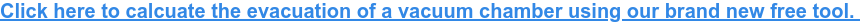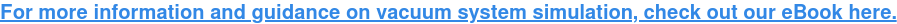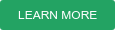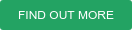The evacuation of a vacuum chamber to a given target pressure is a common application for vacuum pumps – but as our article on Vacuum Simulation Calculation points out, the calculation of vacuum applications is a challenging task.

To calculate the evacuation time for a vacuum chamber, numerous physical factors must be taken into account, i.e. conductance, thermal effects, pumping speed, etc. For a quick estimate, however, it is often sufficient to perform a segmented use of the following formula:

t = (V / S)  * ln(p1 / p2)

This formula gives you the ideal evacuation time which, in reality, will be longer depending on factors described later. You can also estimate the minimum required pumping speed (S) for a required evacuation time (t) by using:

S = (V / t)  * ln(p1 / p2)

We'll explain this calculation in detail later on. If you want to see it in action, you can use our evacuation tool to see the evacuation performance for a given speed curve:Please note that these results can only be regarded as a good estimate for rough vacuum and fine vacuum pressure ranges from 1000 mbar to approx. 0,1 mbar. For a more accurate vacuum system calcualtion, we recommend that you contact us or use a more sophisticated calculation tool such as on https://calc.leybold.com.

## Calculation basics

As we mentioned earlier, we can use the simple logarithmic equation below to calculate estimated evacuation times:

t = (V / S) * ln(p1 / p2)

where V is the volume of the vessel, S is the pumping speed and p1, p2 represent the start and target pressure respectively. However, the problem with this simple approach is that various factos cannot be taken into account. These are listed below.

## Pumping speed

Using the equation, a constant suction speed is assumed. To make a more accurate calculation, we apply this equation on multiple segments of the typical speed curve of the pump type chosen. You can select either oil-lubricated rotary vane pumps or dry scroll, screw, and multistage roots pumps.

## Conductance

In almost all real vacuum applications we have to consider the physical effects of pipe works, valves and other components in the system. Each component connected between a pump and vacuum vessel will reduce the effective pumping speed and result in a longer evacuation time. The smaller the diameter and the longer the pipe, the lower the conductance. Please refer to page 8 of our eBook: 'Fundamentals of Vacuum Generation' for more information. Also bear in mind that vacuum pipes add volume to the system, so they have to be evacuated too!## Thermal effects

Taking gas mass out of a volume changes it's internal energy and thus the temperature of the remaining gas. In slow evacuation processes this temperature drop will be compensated by a heat transfer from the chamber walls. But if the process is fast (less than a few minutes, depending on the chamber dimensions), the gas which has to be pumped will gradually cool, reducing the effective pumping speed of the system.

## Desorption

All materials in a vacuum release gases from their surface. The main component is water vapour, but lubricants are also degassing sources. Our basic calculation tool does not take this into account. Desorption is the dominant source of gas in high and ultra-high vacuum. For more information, see page 13 of our eBook: 'Fundamentals of Vacuum Generation'. Furthermore, the online tool  https://calc.leybold.com allows to enter desorption rates for a more accurate calculation.

Want to see this in action? Access our free calculation tool here:### Filed Under:

Analytical Instruments, Vacuum Simulation

HOW TO CALCULATE VACUUM CHAMBER EVACUATION